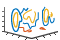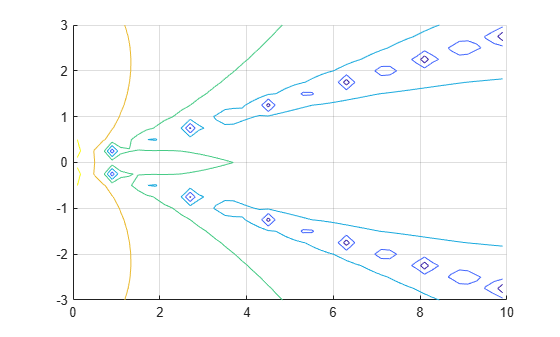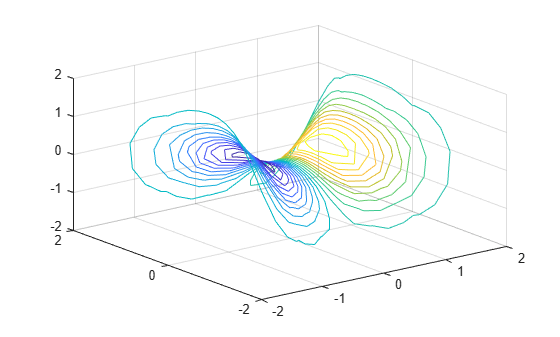# contourslice

Draw contours in volume slice planes

•## Syntax

``contourslice(X,Y,Z,V,xslice,yslice,zslice) ``
``contourslice(V,xslice,yslice,zslice)``
``contourslice(___,num) ``
``contourslice(___,lvls) ``
``contourslice(___,method) ``
``contourslice(ax,___)``
``s = contourslice(___)``

## Description

example

````contourslice(X,Y,Z,V,xslice,yslice,zslice) `draws contours in slices for the volumetric data `V`, where `V` determines the contour colors. Specify `X`,`Y`, and `Z` as the coordinate data. Specify `xslice`, `yslice`, and `zslice` as the slice locations using one of these forms: To draw one or more slice planes that are orthogonal to a particular axis, specify the slice arguments as a scalar or vector.To draw a single slice along a surface, specify all the slice arguments as matrices that define a surface. ```
````contourslice(V,xslice,yslice,zslice)` uses the default coordinate data for `V`. The (x,y,z) location for each element in `V` is based on the column, row, and page index, respectively.```

example

````contourslice(___,num) ` specifies the number of contour lines to draw per slice. Use this option with any of the input arguments from the previous syntaxes.```
````contourslice(___,lvls) ` specifies the values at which to draw contour lines within each slice.```
````contourslice(___,method) ` specifies the interpolation method, where `method` can be `'linear'`, `'cubic'`, or `'nearest'`.```
````contourslice(ax,___)` plots into the specified axes, instead of the current axes (`gca`).```
````s = contourslice(___)` returns the `Patch` objects created.```

## Examples

collapse all

Create a single slice plane through volumetric data and draw contours of the data values.

Create the matrices `X`, `Y`, `Z`, and `V` from the `flow` data set. Draw contours of V in the slice plane where z = 0.

```[X,Y,Z,V] = flow; zslice = 0; contourslice(X,Y,Z,V,[],[],zslice) grid on```Create multiple slice planes through volumetric data. Draw contours of the data values in each of the slice planes. Let MATLAB choose the contour levels.

Create `V` as an array of volume data defined by $v=x{e}^{-{x}^{2}-{y}^{2}-{z}^{2}}$. Draw contours in three slice planes that are orthogonal to the x-axis at the values `-1.2`, `0.8`, and `2`. Do not create any slice planes that are orthogonal to the y-axis or z-axis by specifying empty arrays. Change the axes view to a 3-D view and add grid lines.

```[X,Y,Z] = meshgrid(-2:.2:2); V = X.*exp(-X.^2-Y.^2-Z.^2); xslice = [-1.2,0.8,2]; yslice = []; zslice = []; contourslice(X,Y,Z,V,xslice,yslice,zslice) view(3) grid on```Create multiple slice planes through volumetric data. Draw contours of the data values in each of the slice planes. Specify the levels where you want to draw the contours. Then, add a colorbar.

Draw contours in three slice planes that are orthogonal to the x-axis at the values `-1.2`, `0.8`, and `2`. Draw contours for the volume data between the values `-0.2` and `0.4`, spaced every `0.01`. Add a colorbar to see how the data values map to the contour colors. Also, change the axes view to a 3-D view and add grid lines.

```[X,Y,Z] = meshgrid(-2:.2:2); V = X.*exp(-X.^2-Y.^2-Z.^2); xslice = [-1.2,0.8,2]; lvls = -0.2:0.01:0.4; contourslice(X,Y,Z,V,xslice,[],[],lvls) colorbar view(3) grid on```Create a surface slice through volumetric data and draw contours along the surface of the volume data. Specify the number of contour levels to draw.

Create `V` as an array of volume data defined by $v=x{e}^{-{x}^{2}-{y}^{2}-{z}^{2}}$. Then, show a slice of the volume data along the surface defined by $z={x}^{2}-{y}^{2}$. Draw 20 contours along the surface. Change the axes view to a 3-D view and add grid lines.

```[X,Y,Z] = meshgrid(-5:0.2:5); V = X.*exp(-X.^2-Y.^2-Z.^2); [xsurf,ysurf] = meshgrid(-2:0.2:2); zsurf = xsurf.^2-ysurf.^2; contourslice(X,Y,Z,V,xsurf,ysurf,zsurf,20) view(3) grid on```## Input Arguments

collapse all

x-axis coordinate data, specified as a vector or a 3-D array the same size as `V`. If you specify a 3-D array, it must be monotonic and orthogonally spaced, as if produced by the `meshgrid` function.

Data Types: `single` | `double` | `int8` | `int16` | `int32` | `int64` | `uint8` | `uint16` | `uint32` | `uint64`

y-axis coordinate data, specified as a vector or a 3-D array the same size as `V`. If you specify a 3-D array, it must be monotonic and orthogonally spaced, as if produced by the `meshgrid` function.

Data Types: `single` | `double` | `int8` | `int16` | `int32` | `int64` | `uint8` | `uint16` | `uint32` | `uint64`

z-axis coordinate data, specified as a vector or a 3-D array the same size as `V`. If you specify a 3-D array, it must be monotonic and orthogonally spaced, as if produced by the `meshgrid` function.

Data Types: `single` | `double` | `int8` | `int16` | `int32` | `int64` | `uint8` | `uint16` | `uint32` | `uint64`

Volume data, specified as a 3-D array.

Data Types: `single` | `double` | `int8` | `int16` | `int32` | `int64` | `uint8` | `uint16` | `uint32` | `uint64`

x-axis slice values, specified in one of these forms:

• Scalar — Draw one slice plane orthogonal to the x-axis at the specified location.

• Vector — Draw multiple slice planes orthogonal to the x-axis at the specified locations.

• `[]` — Do not draw any slice planes.

• Matrix — Draw the slice along a surface, instead of a plane. If you use this option, then `yslice` and `zslice` also must be matrices that are the same size.

Example: `[2 4]` draws slice planes orthogonal to the x-axis at the values 2 and 4.

Data Types: `single` | `double` | `int8` | `int16` | `int32` | `int64` | `uint8` | `uint16` | `uint32` | `uint64`

y-axis slice values, specified in one of these forms:

• Scalar — Draw one slice plane orthogonal to the y-axis at the specified location.

• Vector — Draw multiple slice planes orthogonal to the y-axis at the specified locations.

• `[]` — Do not draw any slice planes.

• Matrix — Draw the slice along a surface, instead of a plane. If you use this option, then `xslice` and `zslice` also must be matrices that are the same size.

Example: `[2 4]` draws slice planes orthogonal to the y-axis at the values 2 and 4.

Data Types: `single` | `double` | `int8` | `int16` | `int32` | `int64` | `uint8` | `uint16` | `uint32` | `uint64`

z-axis slice values, specified in one of these forms:

• Scalar — Draw one slice plane orthogonal to the z-axis at the specified location.

• Vector — Draw multiple slice planes orthogonal to the z-axis at the specified locations.

• `[]` — Do not draw any slice planes.

• Matrix — Draw the slice along a surface, instead of a plane. If you use this option, then `xslice` and `yslice` also must be matrices that are the same size.

Example: `[2 4]` draws slice planes orthogonal to the z-axis at the values 2 and 4.

Data Types: `single` | `double` | `int8` | `int16` | `int32` | `int64` | `uint8` | `uint16` | `uint32` | `uint64`

Number of contour lines per slice, specified as a scalar.

Example: `4` draws four contour lines within each slice.

Data Types: `single` | `double` | `int8` | `int16` | `int32` | `int64` | `uint8` | `uint16` | `uint32` | `uint64`

Contour line levels, specified as a vector. If you want to draw a single contour line per slice at a particular level, then specify the vector in the form `[lvl lvl]`.

Example: `[1 3 5]` draws contour lines at the levels 1, 3, and 5 within each slice.

Example: `[4 4]` draws a single contour line at the level 4 within each slice.

Data Types: `single` | `double` | `int8` | `int16` | `int32` | `int64` | `uint8` | `uint16` | `uint32` | `uint64`

Interpolation method, specified as one of these values:

• `'linear'` — Linear interpolation of the values at neighboring grid points in each respective dimension. This value is the default value when the slice is a surface.

• `'cubic'` — Cubic interpolation of the values at neighboring grid points in each respective dimension.

• `'nearest'` — Nearest grid point value. This value is the default value when the slices are planes.

Target axes, specified as an `Axes` object. If you do not specify the axes, then `contourslice` plots into the current axes.

## Algorithms

The `contourslice` function does not clear existing plots before plotting.

## Version History

Introduced before R2006a